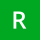Home Communities
IT Knowledge
Inspiration
Languages
EN

# JavaScript - example, how to calculate logarithm with custom base?

4 points
Created by:Reilly-Collier
800

In this article, we would like to show how to calculate logarithm for specific base using JavaScript.

Practical example:

``````// ONLINE-RUNNER:browser;

function calculateLogarithm(base, x) {
var a = Math.log(x);
var b = Math.log(base);

return a / b;
}

// Logarithm with custom base:
//                               base    x               y
console.log( calculateLogarithm( 2,      2      ) ); //  1
console.log( calculateLogarithm( 2,      4      ) ); //  2
console.log( calculateLogarithm( Math.E, Math.E ) ); //  1
console.log( calculateLogarithm( 3,      9      ) ); // ~2
console.log( calculateLogarithm( 3,      81     ) ); // ~4
console.log( calculateLogarithm( 10,     10     ) ); //  1``````

Note: read this article to see `Math.log()` method documentation.

Join to our subscribers to be up to date with content, news and offers.